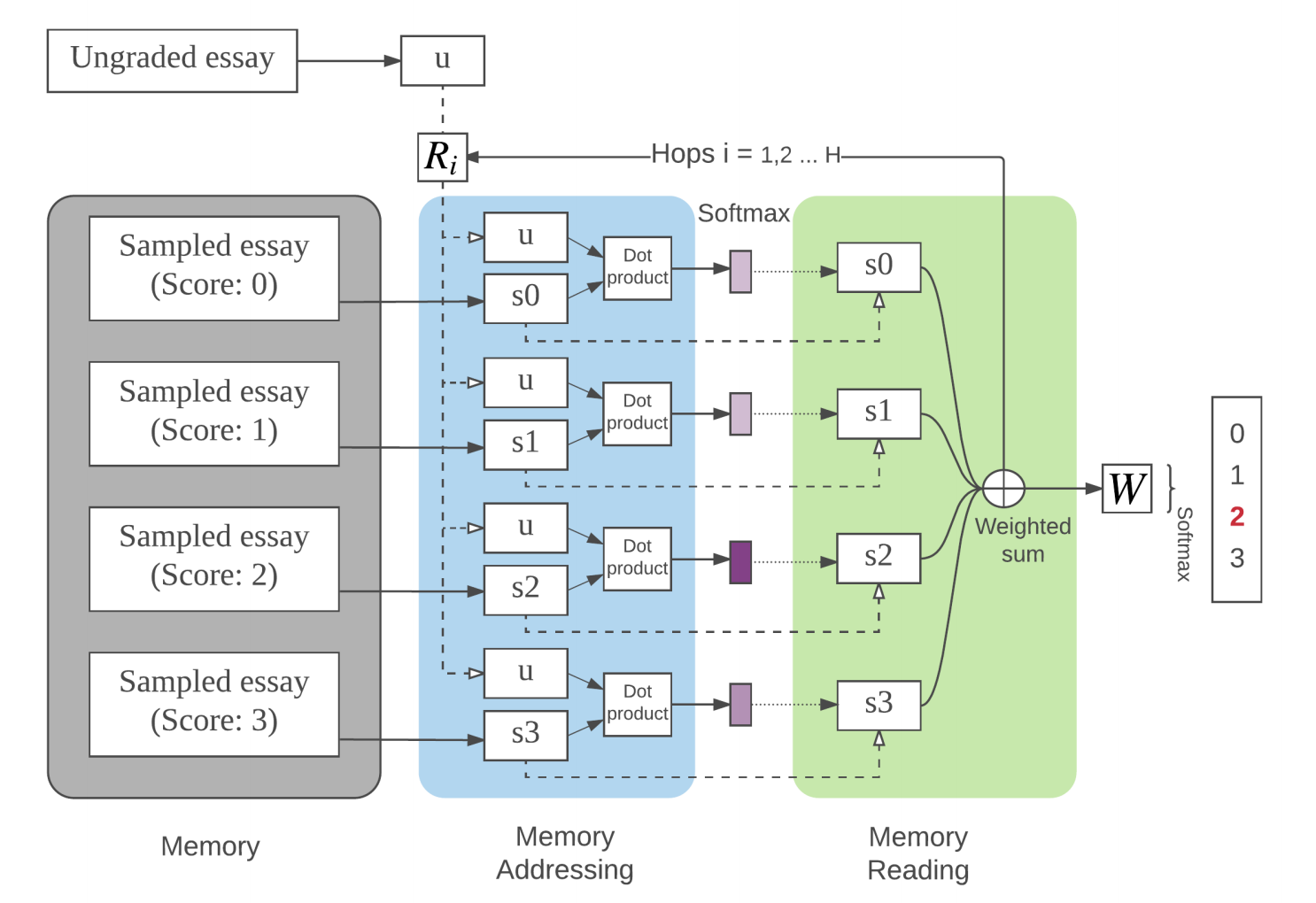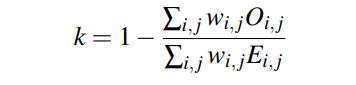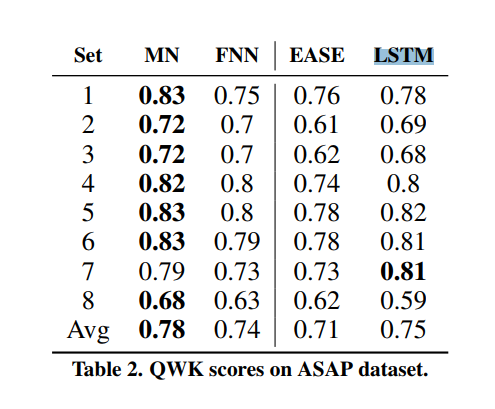### 模型结构• 输入表示层: 将学生作文用向量表示
• 内存寻址层: 将”标准作文”加载进内存, 根据输入的作文, 计算不同的标准作文的权重
• 内存读取层: 根据权重计算”标准作文”的加权之和
• 输出层: 做出预测

### 数学模型

#### 输入层

$$m_i = \sum_j l_j \cdot Wx_{ij}$$

$$l_{kj} = (1− j/J)−(k/d) (1−2 j/J)$$

#### 内存寻址

$$p_i = Softmax(x A^T \cdot m_i B^T)$$

#### 内存读取

$$o = \sum_i p_i m_i C^T$$

#### 多层hops

$$u_2 = Relu(R_1(u_1+o))$$

#### 输出层

$$\hat s = Softmax(u_h W + b)$$

### 评价指标### 基线

• Enhanced AI Scoring Engine (EASE): 开源的AES系统, 它几乎是所有的开源系统中QWK得分最高的
• a simple multi-layer forward neural networks (FNN) model
• LSTM

### 结果### 参考文献

1. Hongbo Chen and Ben He. 2013. Automated Essay
Scoring by Maximizing Human-Machine Agreement. In
EMNLP.

2. Jeffrey Pennington, Richard Socher, and Christopher D
Manning. 2014. Glove: Global Vectors for Word
Representation. In EMNLP, Vol. 14. 1532–1543.

3. Peter Phandi, Kian Ming Adam Chai, and Hwee Tou Ng. 2015. Flexible Domain Adaptation for Automated Essay
Scoring Using Correlated Linear Regression. In EMNLP.

4. Sainbayar Sukhbaatar, Arthur Szlam, Jason Weston, and
Rob Fergus. 2015. End-To-End Memory Networks. In
Advances in Neural Information Processing Systems 28,
C Cortes, N D Lawrence, D D Lee, M Sugiyama, and
R Garnett (Eds.). Curran Associates, Inc., 2440–2448.

5. Kaveh Taghipour and Hwee Tou Ng. 2016. A Neural
Approach to Automated Essay Scoring. In EMNLP.

6. Jason Weston, Sumit Chopra, and Antoine Bordes. 2014.
Memory Networks. CoRR abs/1410.3916 (2014).

### 赞助